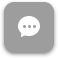Call Now 855-300-1469

# MTH 1701 College Algebra

#### Course Description

Real-number system; arithmetic operations with polynomials, special products and factoring; linear, fractional and quadratic equations; inequalities, exponents, radicals and absolute values; functions and graphs; and complex numbers, logarithms, logarithmic and exponential functions. Credit cannot be applied toward any Florida Tech degree except business, communication, humanities, management and psychology.

#### Course Objectives

Upon completion of this course, students should be able to

• Plot points and equations and interpret information using the rectangular coordinate system.  (This would include finding equations of lines, parallel lines, and perpendicular lines.)
• Solve linear and rational equations in one variable.
• Use mathematical equations to model real-life problems.
• Perform operations with real and complex numbers.
• Solve quadratic equations by factoring, completing the square, and by the quadratic formula.
• Solve radical equations, equations with rational exponents, and equations involving absolute value.
• Solve inequalities of the same types as in Objective 6.
• Use function notation and identify the domain and range.
• Perform operations on functions including finding the inverse and composition of two functions.
• Use properties and perform operations on logarithmic and exponential functions.
• Solve systems of linear equations in two or three variables.
• Perform partial fraction decomposition of rational expressions.
• Solve systems of inequalities and graph the solutions.

### Week 8

##### Lecture: Course Conclusion and Final Review

The course description, objectives and learning outcomes are subject to change without notice based on enhancements made to the course. March 2012